Courses

# Notes | EduRev

## Class 10 : Notes | EduRev

The document Notes | EduRev is a part of the Class 10 Course Class 10 Mathematics by VP Classes.
All you need of Class 10 at this link: Class 10

Ques 1: The ages of two friends Ani and Biju differ by 3 years. Ani’s father Dharam is twice as old as Ani and Biju is twice as old as his sister Cathy. The ages of Cathy and Dharam differ by 30 years. Find the ages of Ani and Biju.
Sol: Let the age of Ani = x years
And the age of Biju = y years
Case I:
y > x
According to 1st condition:
y - x = 3     ...(1)
∵ [Age of Ani’s father] = 2 [Age of Ani]
∴ = 2x years
Also, [Age of Biju’s sister]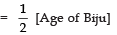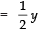According to IInd condition: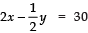⇒ 4x − y = 60      ...(2)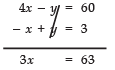⇒ x = 63/3 = 21
From (1), y - 21 = 3  ⇒  y = 3 + 21 = 24
∴ Age of Ani = 21 years
Age of Biju = 24 years
Case II:
x > y
∴ x - y = 3     ...(1)
According to the condition: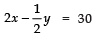⇒ 4x − y = 60     ...(2)
Subtracting (1) from (2),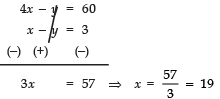From (1), 19 - y = 3
⇒ - y = 3 - 19
⇒ - y = - 16  ⇒  y = 16
⇒ Age of Ani = 19 years
Age of Biju = 16 years.

Ques 2: One says, ‘‘Give me a hundred, friend! I shall then become twice as rich as you’’. The other replies, ‘‘If you give me ten, I shall be six times as rich as you’’. Tell me what is the amount of their (respective) capital ? [From the Bijaganita of Bhaskara II]
[Hint: x + 100 = 2 (y - 100), y + 10 = 6 (x - 10)].
Sol: Let the capital of 1st friend = ₹ x
And capital of 2nd friend = ₹ y
According to the condition,
x + 100 = 2 (y - 100)
⇒ x + 100 - 2y + 200 = 0
⇒ x - 2y + 300 = 0     ...(1)
And 6 (x - 10) = y + 10
⇒ 6x - 60 - y - 10 = 0
⇒ 6x - y - 70 = 0    ...(2)
From (1), x = - 300 + 2y
From (2), 6x - y - 70 = 0
⇒ 6 [- 300 + 2y] - y - 70 = 0
⇒ - 1800 + 12y - y - 70 = 0
⇒ - 1870 + 11y = 0
⇒ y = 1870/11= 170
⇒ y = 1870/11 = 170
Now, x = - 300 + 2y
= - 300 + 2 (170)
= - 300 + 340
= 40
Thus, 1st friend has ₹ 40 and the 2nd friend has ₹ 170.

Ques 3: A train covered a certain distance at a uniform speed. If the train would have been 10 km/h faster, it would have taken 2 hours less than the scheduled time. And, if the train were slower by 10 km/h; it would have taken 3 hours more than the scheduled time. Find the distance covered by the train.
Sol: Let the actual speed of the train = x km/hr
And the actual time taken = y hours
∵ Distance = speed × time
1st Condition:
(x + 10) × (y - 2) = xy
⇒ xy - 2x + 10y - 20 = xy
⇒ 2x - 10y + 20 = 0    ...(1)
2nd Condition:
(x - 10) × (y + 3) = xy
⇒ xy + 3x - 10y - 30 = xy
⇒ 3x - 10y - 30 = 0 ...(2)
Using cross multiplication for solving (1) and (2):
a1 = 2,  b1 = - 10,  c1 = 20
a2 = 3,  b2 = - 10,  c2 = - 30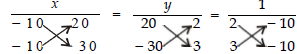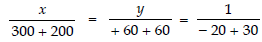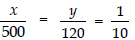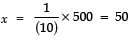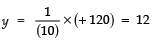Thus, the distance covered by the train = 50 × 12 km = 600 km.

Ques 4: The students of a class are made to stand in rows. If 3 students are extra in a row, there would be 1 row less. If 3 students are less in a row, there would be 2 rows more. Find the number of students in the class.
Sol: Let the number of students = x
And the number of rows = y
∴ Number of students in each row =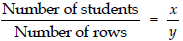1st Condition:
=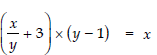[Number of students in a row × Number of rows = Number of students]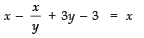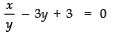...(1)
2nd Condition: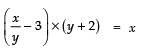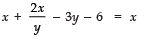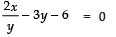...(2)
Let x/y = p
∴ Equations (1) and (2) can be expressed as
p - 3y + 3 = 0 ...(3)
2p - 3y - 6 = 0 ...(4)
Subtracting (3) from (4), we get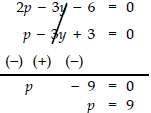From (3), we get
9 − 3y + 3 = 0 ⇒− 3y = − 12
⇒ y = -12/-3 = 4
Since, x /y = 9   [∵ p = 9]
x/4 = 9 ⇒ x = 4 × 9 = 36
Thus, number of students in the class
= xy
= 36 × 4 = 144.

Ques 5: In a Δ ABC, ∠C = 3 ∠B = 2 (∠A + ∠B). Find the three angles.
Sol: ∵ Sum of angles of a triangle = 180°
∴ ∠A + ∠B + ∠C = 180° ...(1)
∵ ∠C = 3 ∠B = 2 (∠A + ∠B) ...(2)
From (1) and (2), we have:
∠A + ∠B + 2 (∠A + ∠B) = 180°
⇒ ∠A + ∠B + 2 ∠A + 2 ∠B = 180°
⇒ 3 ∠A + 3 ∠B = 180°
⇒ ∠A + ∠B = 60 ...(3)
Also,
∠A + ∠B + 3 ∠B = 180°
⇒ ∠A + 4 ∠B = 180°   ...(4)
Subtracting (3) from (4),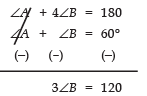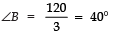From (4),
∠A + 4 (40) = 180°  ⇒  ∠A = 180° - (4 × 40)
⇒ ∠A = 180° - 160 = 20°
Again ∠C = 3 ∠B = 3 × 40° = 120°
Thus, ∠A = 20°,  ∠B = 40°  and  ∠C = 120°.

Ques 6: Draw the graphs of the equations 5x - y = 5 and 3x - y = 3. Determine the co-ordinates of the vertices of the triangle formed by these lines and the y-axis.
Sol:
To draw the graph of 5x - y = 5, we get
5x - y = 5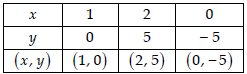and for equation 3x - y = 3, we get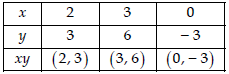Plotting the points (1, 0), (2, 5) and (0, - 5), we get a straight line l1. Plotting the points (2, 3), (3, 6) and (0, - 3) we get a straight line l2.
From the figure, obviously, the vertices of the triangle formed are :
A (1, 0), B (0, - 5) and C (0, - 3).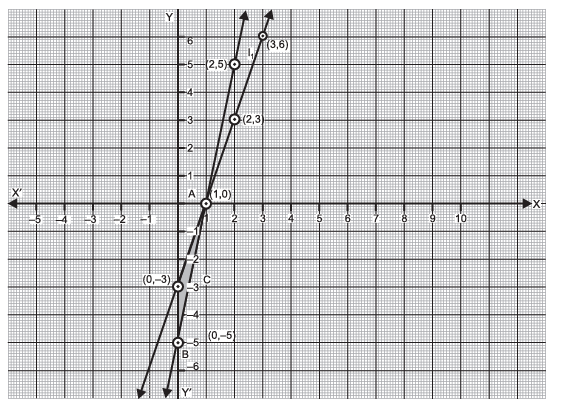Ques 7: Solve the following pair of linear equations:
(i) px + qy = p - q
qx - py = p + q

(ii) ax + by = c
bx + ay = 1 + c

(iii)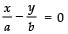ax + by = a2 + b2

(iv) (a - b) x + (a + b) y = a2 - 2ab - b2
(a + b) (x + y) = a2 + b2

(v) 152x - 378y = - 74
- 378x + 152y = - 604
Sol: (i) We have:
px + qy = p - q   ...(1)
qx - py = p + q    ...(2)
Multiply (1) by p and (2) by q, and adding: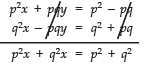⇒ (p2 + q2) x = p2 + q2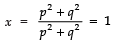From (1),
p (1) + q y = p − q
⇒ p + q y = p − q
⇒ q y = p − q − p = q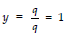Thus, the required solution is: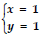(ii) We have:
ax + by = c     ...(1)
bx + ay = (1 + c)   ...(2)
By cross multiplication, we have:
A1 = a,  B1 = b,  C1 = - c
A2 = b,  B2 = a,  C2 = - (1 + c)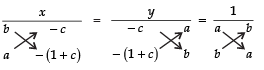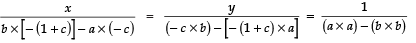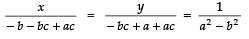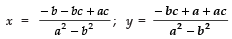or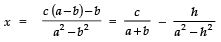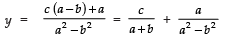(iii) We have:
=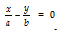...(1)
ax + by = a2 + b2      ...(2)
From (1), we have: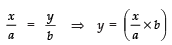Substituting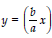in (2), we have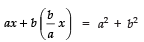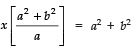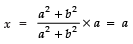⇒ x = a
Substituting x = a in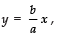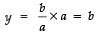⇒ x = b
Thus, the required solution is
x = a
y = b
(iv) We have:
(a - b) x + (a + b) y = a2 - 2ab - b2   ...(1)
(a + b) (x + y) = a2 + b2    ...(2)
From (2),
(a + b) x + (a + b) y = a2 + b2 ...(3)
Subtracting (3) from (1), we get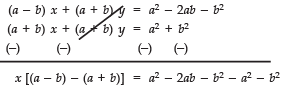⇒ x [a − b − a − b]= − 2ab − 2b2
⇒ x (− 2b)= − 2b (a + b)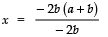⇒ x =(a + b
Substituting x = (a + b) in (1),
(a - b) (a + b) + (a + b) y = a2 - 2ab - b2
⇒ a2 - b2 + (a + b) y = a2 - 2ab - b2
⇒ (a + b) y = a2 - 2ab - b2 - a2 + b2
⇒ (a + b) y = - 2ab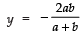Thus, x = a + b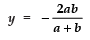Thus,
(v) We have:
152x - 378y = - 74     ...(1)
- 378x + 152y = - 604  ...(2)
Adding (1) and (2), we have:
- 226x - 226y = - 678
⇒ x + y = 3     ...(3)
[Dividing throughout by - 226]
Subtracting (1) from (2),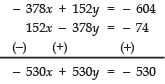⇒− x + y = − 1
⇒ x − y = 1     ...(4)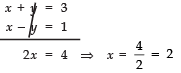Subtracting (3) from (4),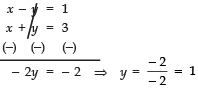Thus, the required solution is
x = 2  and  y = 1

Ques 8: ABCD is a cyclic quadrilateral (see figure.). Find the angles of the cyclic quadrilateral.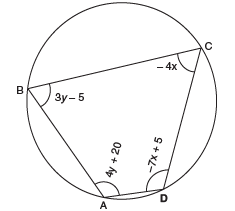Sol: ∵ ABCD is a cyclic quadrilateral.
∴  ∠A + ∠C = 180°  and  ∠B + ∠D = 180°
⇒ [4y + 20] + [- 4x] = 180°
⇒ 4y - 4x + 20° - 180° = 0
⇒ 4y - 4x - 160° = 0
⇒ y - x - 40° = 0      ...(1)
[Dividing throughout by 4]
And
[3y - 5] + [- 7x + 5] = 180°
⇒ 3y - 5 + 5 - 7x - 180° = 0
⇒ 3y - 7x - 180° = 0 ...(2)
Multiplying (1) by 7 and subtracting from (2),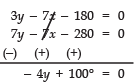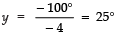Now, substituting y = 25° in (1)
y - x = 40  ⇒  - x = 40 - y
= 40° - 25° = 15°
⇒ x = - 15°
∴ ∠A = 4y + 20° = 4 (25°) + 20°
= 100° + 20° = 120°
∠B = 3y - 5° = 3 (25°) - 5°
= 75° - 5° = 70°
∠C = - 4x = - (- 15°)
= 60°
∠D = - 7x + 5° = - 7 (- 15) + 5°
= 105° + 5° = 120°
Thus, ∠A = 120°,  ∠B = 70°,  ∠C = 60°,  ∠D = 110°.

Offer running on EduRev: Apply code STAYHOME200 to get INR 200 off on our premium plan EduRev Infinity!

132 docs

,

,

,

,

,

,

,

,

,

,

,

,

,

,

,

,

,

,

,

,

,

;